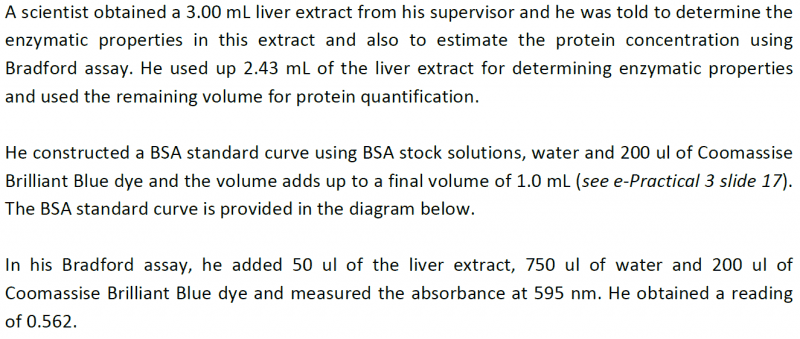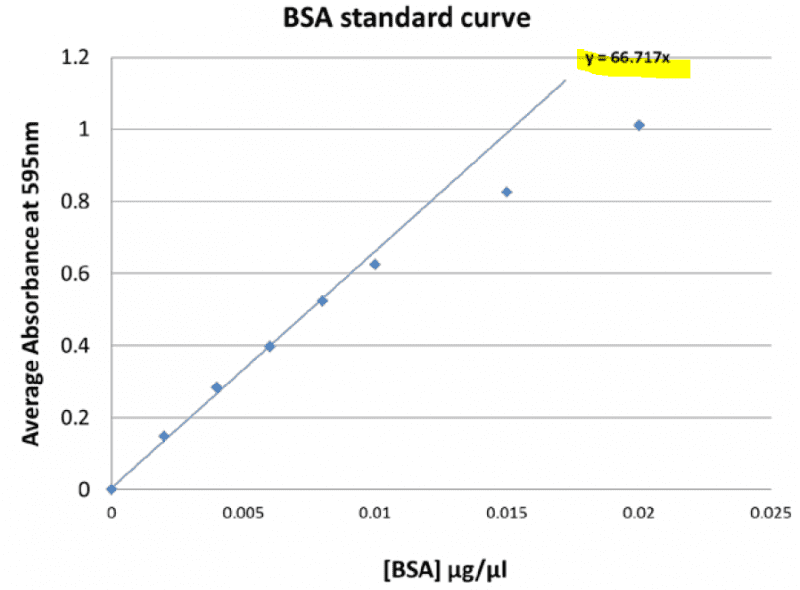## Homework Statement

View attachment 76905
View attachment 76906

## Homework Equations

3. The Attempt at a Solution [/B]
Hi I have a question on Braford Assay:
I am given this question:
I did the caluclation as follows:
y = 66.717x
Since given absorbance = 0.562
0.562 = 66.717x
x = 0.00842ug/ul
But the answers given is 0.168mg/ml
My answer and the correct answer differ by a factor of 20? Why is that so?

Last edited by a moderator:

Borek
Mentor
My answer and the correct answer differ by a factor of 20?

0.562 is not absorbance on the original solution.

epenguin
Homework Helper
Gold Member
Yes, I was trying to answer this question but when I press your links this time I am told I don't have right to access the page. But I remember when I saw it before there was something about an 0.05 ml sample - which is 1/20 of an ml. Could these occurrences of 1/20 be in any easy connected?

I still dont get it though. Sorry. I will post the image again, Sorry for the trouble pal!
Anyway, even it is a factor of 20. My answer has a unit of ug/ul and the correct answer is in mg/ml so technically it is not a factor of 20??Borek
Mentor
Please reread the last paragraph of the attached text, it clearly tells you why the difference is 20.

50+750+200=1000.

I am suppose to find the protein concentration of the liver sample what do i do with the 1000ul ?
Is this correct?
Since x = 0.00842ug/ul and total volume of my assay --> 1000 ul
Then mass = 0.00842 * 1000 = 8.42 ug
Concentration of protein = 8.42ug/50ul (of protein in liver)
= 0.1684 ug/ul = 0.1684 mg/ml

Borek
Mentor
Correct.

50 μl of your sample were diluted 20 times to 1000μl.

Thank you!